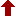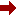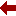#### SLIM21

 Sort Order Chronological Author Title ItemType,Author ItemType,Title Display Format Tabular Publication Bibliography Items / Page 5 10 25

Click the serial number on the left to view the details of the item.
#  AuthorTitleAccn#YearItem Type Claims
1 Levi, Decio Symmetries and Integrability of Difference Equations I10153 2017 eBook
2 Maximon, Leonard C Differential and Difference Equations I09655 2016 eBook
3 Akhmet, Marat Bifurcation in Autonomous and Nonautonomous Differential Equations with Discontinuities I08930 2017 eBook
4 Akhmet, Marat Replication of Chaos in Neural Networks, Economics and Physics I08638 2016 eBook
5 Orlandi, Paolo Fluid Flow Phenomena I11112 2000 eBook
6 Luo, Albert C. J Discretization and Implicit Mapping Dynamics I07463 2015 eBook
7 Dong, Shi-Hai Wave Equations in Higher Dimensions I07415 2011 eBook
9 Ronald E. Mickens Difference equations: Theory, Applications and Advanced Topics 025996 2015 Book
10 Zafar Ahsan Mathematical methods 023422 2009 Book
 (page:1 / 2) [#13] Next Page Last Page

1.TitleSymmetries and Integrability of Difference Equations : Lecture Notes of the Abecederian School of SIDE 12, Montreal 2016
Author(s)Levi, Decio;Rebelo, Rapha??l;Winternitz, Pavel
PublicationCham, Springer International Publishing, 2017.
DescriptionX, 435 p. 67 illus., 26 illus. in color : online resource
Abstract NoteThis book shows how Lie group and integrability techniques, originally developed for differential equations, have been adapted to the case of difference equations. Difference equations are playing an increasingly important role in the natural sciences. Indeed, many phenomena are inherently discrete and thus naturally described by difference equations. More fundamentally, in subatomic physics, space-time may actually be discrete. Differential equations would then just be approximations of more basic discrete ones. Moreover, when using differential equations to analyze continuous processes, it is often necessary to resort to numerical methods. This always involves a discretization of the differential equations involved, thus replacing them by difference ones. Each of the nine peer-reviewed chapters in this volume serves as a self-contained treatment of a topic, containing introductory material as well as the latest research results and exercises. Each chapter is presented by one or more early career researchers in the specific field of their expertise and, in turn, written for early career researchers. As a survey of the current state of the art, this book will serve as a valuable reference and is particularly well suited as an introduction to the field of symmetries and integrability of difference equations. Therefore, the book will be welcomed by advanced undergraduate and graduate students as well as by more advanced researchers
ISBN,Price9783319566665
Keyword(s)1. ALGEBRA 2. Difference and Functional Equations 3. DIFFERENCE EQUATIONS 4. EBOOK 5. EBOOK - SPRINGER 6. Field theory (Physics) 7. Field Theory and Polynomials 8. FUNCTIONAL EQUATIONS 9. Numerical and Computational Physics, Simulation 10. PHYSICS
Item TypeeBook
Circulation Data
 Accession# Call# Status Issued To Return Due On Physical Location I10153 On Shelf

2.TitleDifferential and Difference Equations : A Comparison of Methods of Solution
Author(s)Maximon, Leonard C
PublicationCham, Springer International Publishing, 2016.
DescriptionXV, 162 p : online resource
Abstract NoteThis book, intended for researchers and graduate students in physics, applied mathematics and engineering, presents a detailed comparison of the important methods of solution for linear differential and difference equations - variation of constants, reduction of order, Laplace transforms and generating functions - bringing out the similarities as well as the significant differences in the respective analyses. Equations of arbitrary order are studied, followed by a detailed analysis for equations of first and second order. Equations with polynomial coefficients are considered and explicit solutions for equations with linear coefficients are given, showing significant differences in the functional form of solutions of differential equations from those of difference equations. An alternative method of solution involving transformation of both the dependent and independent variables is given for both differential and difference equations. A comprehensive, detailed treatment of Green???s functions and the associated initial and boundary conditions is presented for differential and difference equations of both arbitrary and second order. A dictionary of difference equations with polynomial coefficients provides a unique compilation of second order difference equations obeyed by the special functions of mathematical physics. Appendices augmenting the text include, in particular, a proof of Cramer???s rule, a detailed consideration of the role of the superposition principal in the Green???s function, and a derivation of the inverse of Laplace transforms and generating functions of particular use in the solution of second order linear differential and difference equations with linear coefficients
ISBN,Price9783319297361
Keyword(s)1. APPLIED MATHEMATICS 2. Difference and Functional Equations 3. DIFFERENCE EQUATIONS 4. DIFFERENTIAL EQUATIONS 5. EBOOK 6. EBOOK - SPRINGER 7. ENGINEERING MATHEMATICS 8. FUNCTIONAL EQUATIONS 9. Mathematical and Computational Engineering 10. Mathematical Applications in the Physical Sciences 11. Mathematical Methods in Physics 12. MATHEMATICAL PHYSICS 13. ORDINARY DIFFERENTIAL EQUATIONS 14. PHYSICS
Item TypeeBook
Circulation Data
 Accession# Call# Status Issued To Return Due On Physical Location I09655 On Shelf

3.TitleBifurcation in Autonomous and Nonautonomous Differential Equations with Discontinuities
Author(s)Akhmet, Marat;Kashkynbayev, Ardak
PublicationSingapore, Springer Singapore, 2017.
DescriptionXI, 166 p. 31 illus., 26 illus. in color : online resource
Abstract NoteThis book is devoted to bifurcation theory for autonomous and nonautonomous differential equations with discontinuities of different types. That is, those with jumps present either in the right-hand-side or in trajectories or in the arguments of solutions of equations. The results obtained in this book can be applied to various fields such as neural networks, brain dynamics, mechanical systems, weather phenomena, population dynamics, etc. Without any doubt, bifurcation theory should be further developed to different types of differential equations. In this sense, the present book will be a leading one in this field. The reader will benefit from the recent results of the theory and will learn in the very concrete way how to apply this theory to differential equations with various types of discontinuity. Moreover, the reader will learn new ways to analyze nonautonomous bifurcation scenarios in these equations. The book will be of a big interest both for ??beginners and experts in the field. For the former group of specialists, that is, undergraduate and graduate students, the book will be useful since it provides a strong impression that bifurcation theory can be developed not only for discrete and continuous systems, but those which combine these systems in very different ways. The latter group of specialists will find in this book several powerful instruments developed for the theory of discontinuous dynamical systems with variable moments of impacts, differential equations with piecewise constant arguments of generalized type and Filippov systems. A significant benefit of the present book is expected to be for those who consider bifurcations in systems with impulses since they are presumably nonautonomous systems
ISBN,Price9789811031809
Keyword(s)1. Applications of Nonlinear Dynamics and Chaos Theory 2. Control and Systems Theory 3. Control engineering 4. Difference and Functional Equations 5. DIFFERENCE EQUATIONS 6. DIFFERENTIAL EQUATIONS 7. Dynamical Systems and Ergodic Theory 8. DYNAMICS 9. EBOOK 10. EBOOK - SPRINGER 11. ERGODIC THEORY 12. FUNCTIONAL EQUATIONS 13. ORDINARY DIFFERENTIAL EQUATIONS 14. STATISTICAL PHYSICS
Item TypeeBook
Circulation Data
 Accession# Call# Status Issued To Return Due On Physical Location I08930 On Shelf

4.TitleReplication of Chaos in Neural Networks, Economics and Physics
Author(s)Akhmet, Marat;Fen, Mehmet Onur
PublicationBerlin, Heidelberg, Springer Berlin Heidelberg, 2016.
DescriptionXV, 457 p. 141 illus., 133 illus. in color : online resource
Abstract NoteThis book presents detailed descriptions of chaos for continuous-time systems. It is the first-ever book to consider chaos as an input for differential and hybrid equations. Chaotic sets and chaotic functions are used as inputs for systems with attractors: equilibrium points, cycles and tori. The findings strongly suggest that chaos theory can proceed from the theory of differential equations to a higher level than previously thought. The approach selected is conducive to the in-depth analysis of different types of chaos. The appearance of deterministic chaos in neural networks, economics and mechanical systems is discussed theoretically and supported by simulations. As such, the book offers a valuable resource for mathematicians, physicists, engineers and economists studying nonlinear chaotic dynamics
ISBN,Price9783662475003
Keyword(s)1. Applications of Nonlinear Dynamics and Chaos Theory 2. BIOMATHEMATICS 3. Difference and Functional Equations 4. DIFFERENCE EQUATIONS 5. EBOOK 6. EBOOK - SPRINGER 7. ECONOMIC THEORY 8. Economic Theory/Quantitative Economics/Mathematical Methods 9. FUNCTIONAL EQUATIONS 10. Mathematical and Computational Biology 11. MECHANICS 12. Mechanics, Applied 13. STATISTICAL PHYSICS 14. Theoretical and Applied Mechanics
Item TypeeBook
Circulation Data
 Accession# Call# Status Issued To Return Due On Physical Location I08638 On Shelf

5.TitleFluid Flow Phenomena : A Numerical Toolkit
Author(s)Orlandi, Paolo
PublicationDordrecht, Springer Netherlands, 2000.
DescriptionXII, 356 p. 10 illus : online resource
Abstract NoteThis book deals with the simulation of the incompressible Navier-Stokes equations for laminar and turbulent flows. The book is limited to explaining and employing the finite difference method. It furnishes a large number of source codes which permit to play with the Navier-Stokes equations and to understand the complex physics related to fluid mechanics. Numerical simulations are useful tools to understand the complexity of the flows, which often is difficult to derive from laboratory experiments. This book, then, can be very useful to scholars doing laboratory experiments, since they often do not have extra time to study the large variety of numerical methods; furthermore they cannot spend more time in transferring one of the methods into a computer language. By means of numerical simulations, for example, insights into the vorticity field can be obtained which are difficult to obtain by measurements. This book can be used by graduate as well as undergraduate students while reading books on theoretical fluid mechanics; it teaches how to simulate the dynamics of flow fields on personal computers. This will provide a better way of understanding the theory. Two chapters on Large Eddy Simulations have been included, since this is a methodology that in the near future will allow more universal turbulence models for practical applications. The direct simulation of the Navier-Stokes equations (DNS) is simple by finite-differences, that are satisfactory to reproduce the dynamics of turbulent flows. A large part of the book is devoted to the study of homogeneous and wall turbulent flows. In the second chapter the elementary concept of finite difference is given to solve parabolic and elliptical partial differential equations. In successive chapters the 1D, 2D, and 3D Navier-Stokes equations are solved in Cartesian and cylindrical coordinates. Finally, Large Eddy Simulations are performed to check the importance of the subgrid scale models. Results for turbulent and laminar flows are discussed, with particular emphasis on vortex dynamics. This volume will be of interest to graduate students and researchers wanting to compare experiments and numerical simulations, and to workers in the mechanical and aeronautic industries
ISBN,Price9789401142816
Keyword(s)1. Classical and Continuum Physics 2. CLASSICAL MECHANICS 3. Continuum physics 4. Difference and Functional Equations 5. DIFFERENCE EQUATIONS 6. EBOOK 7. EBOOK - SPRINGER 8. FUNCTIONAL EQUATIONS 9. MATHEMATICAL PHYSICS 10. MECHANICS 11. Theoretical, Mathematical and Computational Physics
Item TypeeBook
Circulation Data
 Accession# Call# Status Issued To Return Due On Physical Location I11112 On Shelf

6.TitleDiscretization and Implicit Mapping Dynamics
Author(s)Luo, Albert C. J
PublicationBerlin, Heidelberg, Springer Berlin Heidelberg, 2015.
DescriptionX, 310 p. 46 illus., 26 illus. in color : online resource
Abstract NoteThis unique book presents the discretization of continuous systems and implicit mapping dynamics of periodic motions to chaos in continuous nonlinear systems. The stability and bifurcation theory of fixed points in discrete nonlinear dynamical systems is reviewed, and the explicit and implicit maps of continuous dynamical systems are developed through the single-step and multi-step discretizations. The implicit dynamics of period-m solutions in discrete nonlinear systems are discussed. The book also offers a generalized??approach to finding??analytical and numerical solutions of stable and unstable periodic flows to chaos in nonlinear systems with/without time-delay. The bifurcation trees of periodic motions to chaos in the Duffing oscillator are shown as a sample problem, while the discrete Fourier series of periodic motions and chaos are also presented. The book offers a valuable resource for university students, professors, researchers and engineers in the fields of applied mathematics, physics, mechanics, control systems, and engineering
ISBN,Price9783662472750
Keyword(s)1. Applications of Nonlinear Dynamics and Chaos Theory 2. Difference and Functional Equations 3. DIFFERENCE EQUATIONS 4. DYNAMICAL SYSTEMS 5. DYNAMICS 6. EBOOK 7. EBOOK - SPRINGER 8. FUNCTIONAL EQUATIONS 9. STATISTICAL PHYSICS 10. VIBRATION 11. Vibration, Dynamical Systems, Control
Item TypeeBook
Circulation Data
 Accession# Call# Status Issued To Return Due On Physical Location I07463 On Shelf

7.TitleWave Equations in Higher Dimensions
Author(s)Dong, Shi-Hai
PublicationDordrecht, Springer Netherlands, 2011.
DescriptionXXV, 295 p : online resource
Abstract NoteHigher dimensional theories have attracted much attention because they make it possible to reduce much of physics in a concise, elegant fashion that unifies the two great theories of the 20th century: Quantum Theory and Relativity. This book provides an elementary description of quantum wave equations in higher dimensions at an advanced level so as to put all current mathematical and physical concepts and techniques at the reader???s disposal. A comprehensive description of quantum wave equations in higher dimensions and their broad range of applications in quantum mechanics is provided, which complements the traditional coverage found in the existing quantum mechanics textbooks and gives scientists a fresh outlook on quantum systems in all branches of physics. In Parts I and II the basic properties of the SO(n) group are reviewed and basic theories and techniques related to wave equations in higher dimensions are introduced. Parts III and IV cover important quantum systems in the framework of non-relativistic and relativistic quantum mechanics in terms of the theories presented in Part II. In particular, the Levinson theorem and the generalized hypervirial theorem in higher dimensions, the Schr??dinger equation with position-dependent mass and the Kaluza-Klein theory in higher dimensions are investigated. In this context, the dependence of the energy levels on the dimension is shown. Finally, Part V contains conclusions, outlooks and an extensive bibliography
ISBN,Price9789400719170
Keyword(s)1. Classical and Quantum Gravitation, Relativity Theory 2. Difference and Functional Equations 3. DIFFERENCE EQUATIONS 4. EBOOK 5. EBOOK - SPRINGER 6. FUNCTIONAL EQUATIONS 7. GRAVITATION 8. QUANTUM PHYSICS
Item TypeeBook
Circulation Data
 Accession# Call# Status Issued To Return Due On Physical Location I07415 On Shelf

8.TitleTheory of third-order differntial equations
PublicationNew Delhi, Springer, 2014.
Descriptionxv, 505p.
Abstract NoteThis book discusses the theory of third-order differential equations. Most of the results are derived from the results obtained for third-order linear homogeneous differential equations with constant coefficients. M. Gregus, in his book written in 1987, only deals with third-order linear differential equations. These findings are old, and new techniques have since been developed and new results obtained.
Contents NoteChapter 1 introduces the results for oscillation and non-oscillation of solutions of third-order linear differential equations with constant coefficients, and a brief introduction to delay differential equations is given. The oscillation and asymptotic behavior of non-oscillatory solutions of homogeneous third-order linear differential equations with variable coefficients are discussed in Ch. 2. The results are extended to third-order linear non-homogeneous equations in Ch. 3, while Ch. 4 explains the oscillation and non-oscillation results for homogeneous third-order nonlinear differential equations. Chapter 5 deals with the z-type oscillation and non-oscillation of third-order nonlinear and non-homogeneous differential equations. Chapter 6 is devoted to the study of third-order delay differential equations. Chapter 7 explains the stability of solutions of third-order equations. Some knowledge of differential equations, analysis and algebra is desirable, but not essential, in order to study the topic.
ISBN,Price9788132216131 : Euro 94.99(HB)
Classification517.925
Keyword(s)1. DIFFERENCE EQUATIONS 2. EBOOK 3. EBOOK - SPRINGER 4. NON HOMOGENEOUS EQUATIONS 5. THIRD-ORDER DIFFERENTIAL EQUATIONS
Item TypeBook
Circulation Data
 Accession# Call# Status Issued To Return Due On Physical Location 026048 517.925/PAD/026048 On Shelf I12161 517.925/PAD/ On Shelf

+Copy Specific Information
9.TitleDifference equations: Theory, Applications and Advanced Topics
Author(s)Ronald E. Mickens
Edition3rd
PublicationBoca Raton, CRC Press, 2015.
Descriptionxxii, 533p.
Series(Monographs and Research Notes in Mathematics)
Abstract NoteBook provides a broad introduction to the mathematics of difference equations and some of their applications. Many worked examples illustrate how to calculate both exact and approximate solutions to special classes of difference equations. Along with adding several advanced topics, this edition continues to cover general, linear, first-, second-, and n-th order difference equations; nonlinear equations that may be reduced to linear equations; and partial difference equations. Suitable for self-study or as the main text for courses on difference equations, this book helps readers understand the fundamental concepts and procedures of difference equations. It uses an informal presentation style, avoiding the minutia of detailed proofs and formal explanations.
ISBN,Price9781482230789 : UKP 76.99(HB)
Classification517.929
Keyword(s)1. CAUCHY-EULER EQUATIONS 2. DIFFERENCE EQUATIONS 3. LAMBERT W-FUNCTIONS 4. LINEAR DIFFERENTIAL EQUATIONS 5. NONLINEAR DIFFERENCE EQUATIONS
Item TypeBook

Circulation Data
 Accession# Call# Status Issued To Return Due On Physical Location 025996 517.929/MIC/025996 On Shelf

+Copy Specific Information
10.TitleMathematical methods
Author(s)Zafar Ahsan;Nikhat Ahsan
PublicationNew Delhi, Anamaya Publishers, 2009.
Descriptionx, 332p.
ISBN,Price8188342475 : Rs. 350.00(PB)
Classification51
Keyword(s)1. COMPLEX NUMBERS 2. DIFFERENCE EQUATIONS 3. DIFFERENTIATION 4. FUNCTIONS 5. INTEGRATION 6. MATHEMATICS 7. MATRICES 8. PARTIAL DIFFERENTIAL EQUATIONS 9. QUADRATIC EQUATIONS 10. VECTOR ANALYSIS
Item TypeBook

Circulation Data
 Accession# Call# Status Issued To Return Due On Physical Location 023422 51/AHS/023422 On Shelf

+Copy Specific Information
 (page:1 / 2) [#13] Next Page Last Page